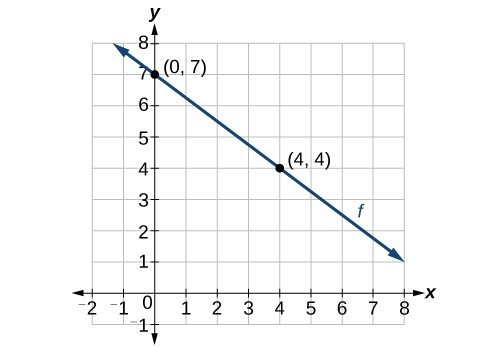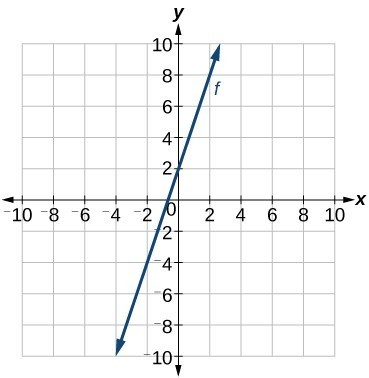## Write the Equation of a Linear Function Part II

### Learning Outcomes

• Write the equation of a linear function from a graph
• Write the equation for a linear function from slope given two points

Now that we have written equations for linear functions using both slope-intercept form and point-slope form, we can choose which method to use based on the information we are given. That information may be provided in the form of a graph, a point and a slope, two points, and so on. Look at the graph of the function f below.We are not given the slope of the line, but we can choose any two points on the line to find the slope. Choose $(0, 7)$ and $(4, 4)$. We can use these points to calculate the slope.

$\begin{array}{l} m & =\dfrac{{y}_{2}-{y}_{1}}{{x}_{2}-{x}_{1}}\hfill \\ & =\dfrac{4 - 7}{4 - 0}\hfill \\ & =-\dfrac{3}{4} \end{array}$

Now we can substitute the slope and the coordinates of one of the points into point-slope form.

$\begin{array}{l}y-{y}_{1}=m\left(x-{x}_{1}\right)\hfill \\ y - 4=-\dfrac{3}{4}\left(x - 4\right)\hfill \end{array}$

If we want to rewrite the equation in slope-intercept form, we would do the following:

$\begin{array}{l}y - 4=-\dfrac{3}{4}\left(x - 4\right)\hfill \\ y - 4=-\dfrac{3}{4}x+3\hfill \\ \text{ }y=-\dfrac{3}{4}x+7\hfill \end{array}$If we wanted to find the equation of the line in slope-intercept form without first using point-slope form, we could have recognized that the line crosses the y-axis when the output value is $7$. Therefore, b = $7$. We now have the initial value b and the slope so we can substitute m and b into slope-intercept form of a line.

The function is $f\left(x\right)=-\dfrac{3}{4}x+7$, and the linear equation would be $y=-\dfrac{3}{4}x+7$.

### How To: Given the graph of a linear function, write an equation to represent the function

1. Identify two points on the line.
2. Use the two points to calculate the slope.
3. Determine where the line crosses the y-axis to identify the y-intercept by visual inspection.
4. Substitute the slope and y-intercept into slope-intercept form of a linear equation.

### Example

Write an equation for a linear function f given its graph shown below.In the following video, we show an example of how to write the equation of a line given its graph.

### Example

If f is a linear function, with $f\left(3\right)=-2$ , and $f\left(8\right)=1$, find an equation for the function in slope-intercept form.

In the video we show another example of how to write a linear function given two points written with function notation.

## Summary

• Given a graph, you can write the equation of the line it represents.
• This method only works for graphs that have points that are easy to verify by visual inspection.